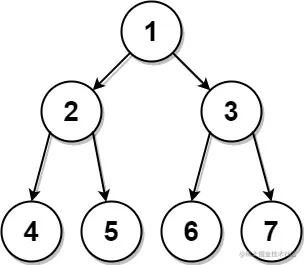# leetcode 889. Construct Binary Tree from Preorder and Postorder Traversal（python）

2022-01-31 23:43:11

「 This is my participation 11 The fourth of the yuegengwen challenge 20 God , Check out the activity details ：2021 One last more challenge

### describe

Given two integer arrays, preorder and postorder where preorder is the preorder traversal of a binary tree of distinct values and postorder is the postorder traversal of the same tree, reconstruct and return the binary tree.

If there exist multiple answers, you can return any of them.

Example 1:``````Input: preorder = [1,2,4,5,3,6,7], postorder = [4,5,2,6,7,3,1]
Output: [1,2,3,4,5,6,7]
Copy code ``````

Example 2:

``````Input: preorder = , postorder = 
Output: 
Copy code ``````

Note:

``````1 <= preorder.length <= 30
1 <= preorder[i] <= preorder.length
All the values of preorder are unique.
postorder.length == preorder.length
1 <= postorder[i] <= postorder.length
All the values of postorder are unique.
It is guaranteed that preorder and postorder are the preorder traversal and postorder traversal of the same binary tree.
Copy code ``````

### analysis

According to the meaning , Given two arrays of integers ,preorder and postorder , among preorder Is the preorder traversal of binary trees with different values , postorder Is the post order traversal of the same tree , Reconstruct and return the binary tree . If there are multiple answers , Request can return any of them . When encountering the problem of tree, directly traverse the depth first DFS Can solve all kinds of difficult and miscellaneous diseases .

Use one preorder And a postorder To reconstruct and restore a binary tree, we need to find the law , From an example, we can see ：

• The root node is postorder Last element of 1 , From the postorder eject
• The root node of the right subtree is 3 , Its presence preorder The index for i , The node of the right subtree contains preorder[i:]
• The nodes of the left subtree contain preorder[1:i]

Continue the above process recursively , You can get the reconstructed binary tree , This question is relatively simple .

``````class TreeNode(object):
def __init__(self, val=0, left=None, right=None):
self.val = val
self.left = left
self.right = right
class Solution(object):
def constructFromPrePost(self, preorder, postorder):
"""
:type preorder: List[int]
:type postorder: List[int]
:rtype: TreeNode
"""
def dfs(pre, post):
if not pre:
return None
if len(pre)==1:
return TreeNode(post.pop())
node = TreeNode(post.pop())
idx = pre.index(post[-1])
node.right = dfs(pre[idx:], post)
node.left = dfs(pre[1:idx], post)
return node
return dfs(preorder, postorder)

Copy code ``````

### Running results

``````Runtime: 40 ms, faster than 75.96% of Python online submissions for Construct Binary Tree from Preorder and Postorder Traversal.
Memory Usage: 13.8 MB, less than 5.77% of Python online submissions for Construct Binary Tree from Preorder and Postorder Traversal.
Copy code ``````